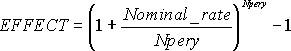Use

The NOMINAL function is one of the Financial functions, it calculates the nominal interest rate of a bond having an effective interest rate and compounding periods per year.

Syntax

NOMINAL(effect_rate, npery)

The NOMINAL function has the following arguments:

effect_rate – Required. The effective interest rate.

npery – Required. The number of compounding periods per year.

ExampleResult:To change the format into the percentage, press Alt, H then P.Function Errors

 Error Occurs #VALUE! If either argument is nonnumeric. #NUM! If effect_rate ≤ 0 or if npery < 1.

Related Function

EFFECT function calculates an effective annual interest rate for a loan or an investment if the compounding periods per year along with the nominal interest rate are given.

The relationship between the NOMINAL and EFFECT functions can be understood by the following formula: